# Why TDA and Clustering Are Not The Same Thing

Cluster analysis is a highly developed field within statistics. It takes as input a set X equipped with a distance function, and potentially some other information, and from that produces a partition of the set, i.e. a decomposition of the set into a collection of groups so that (a) the sets in the collection do not overlap and (b) every point in X is contained in some set in the collection. It is extremely useful because it provides a taxonomy of the points in a data set, since it frequently turns out that the groups can be characterized in a coherent and understandable way.

Topological Data Analysis (TDA) uses cluster analysis in building its networks, and builds on cluster analysis to provide additional precision in the taxonomies that are created. Some people conflate cluster analysis and TDA, which is not accurate. The goal of this post is to explain the differences between the methods.

Let’s first understand a simple clustering method, called single linkage clustering. We are given a set X equipped with a distance function. For simplicity, let’s suppose that it is a set of points in the plane, as pictured below.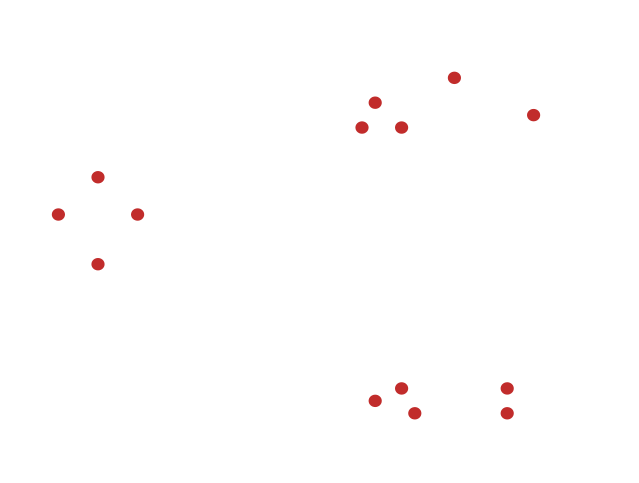The single linkage strategy is to select a threshold real number R, draw edges between any two points whose distance is less than or equal to R, and divide the data set up into the connected components of the resulting network. Here is what happens for a particular choice of the threshold R.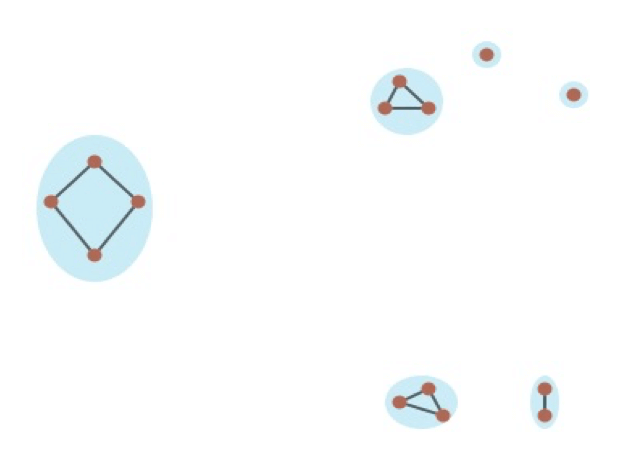The data set is divided up into six groups. Two are singletons. For a slightly larger value of R, we obtain this decomposition.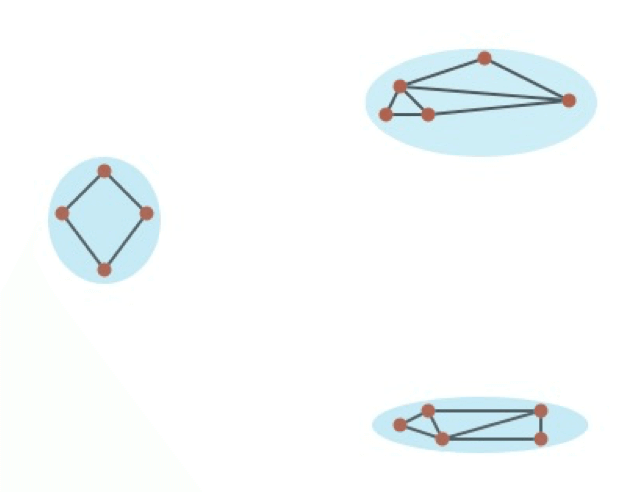With an even larger value of R, we would obtain a single cluster. The challenge lies in knowing how to choose the threshold. Some heuristic criteria have been developed, but in general no particular mathematical methodology can be expected to provide the ideal answers in all situations. Statisticians have developed a method to avoid the selection of a threshold by encoding all threshold level behavior using a single object, a so-called dendrogram. The dendrogram for the above situation looks something like this.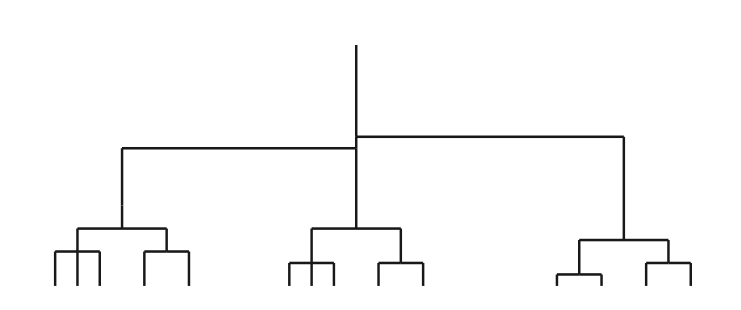This is a mathematical object called a tree. The leaves are the tips of the edges at the bottom of the diagram. Notice that they correspond to the data points – there are 14 of each. The height above the base containing the leaves reflects the clustering threshold R. The correspondence of the data points with the leaves is such that we can identify when two points are merged into the same cluster by tracing the branches until they merge, as indicated in the picture below.

The points a and b are connected at a threshold level slightly below 2. So all the information about the clustering at all thresholds are contained in a single diagram, which is very useful. Note for example that there are three large branches, which correspond to the three main groups visible in the picture of the data points. This diagram therefore gets around the problem of having to select a threshold, and can make clearer the effect of choosing a particular threshold. This method, and variants that also produce dendrograms, get around a very important problem in clustering methodologies, namely the requirement to choose a threshold. As a result, they constitute a large step in the direction of obtaining knowledge about data. There is more to be done, however, as is illustrated by a biomedical example.

The data in this example is a data set consisting of gene expression profiles of breast cancer patients, constructed at the Netherlands Cancer Institute. There are 272 rows, each consisting of expression levels for 1500 genes, and can therefore be regarded as a spreadsheet with 272 rows and 1500 columns, each with a numerical value. The topological network for this data set looks as follows. It comes from the paper .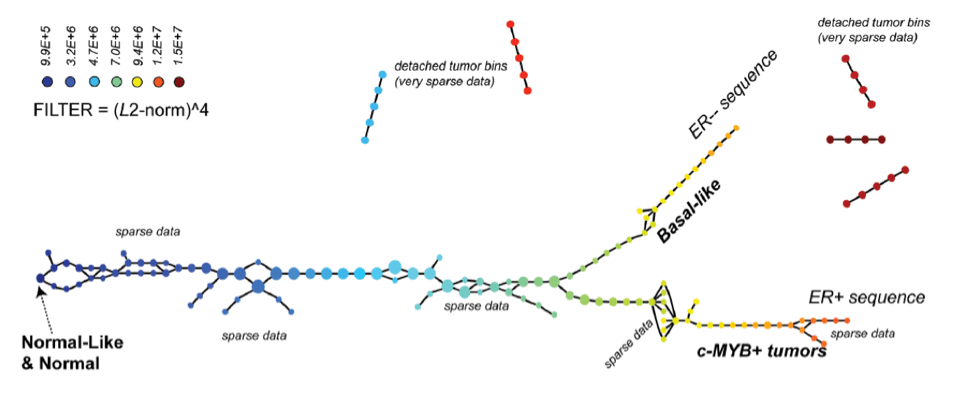The region in the lower right, labelled c-MYB+ tumors, consists entirely of patients who survived the full length of the study. Only gene expression profiling was used to construct the network, without knowledge of any clinical outcomes or judgments. On the other hand, the dendrogram for this data set looks as follows.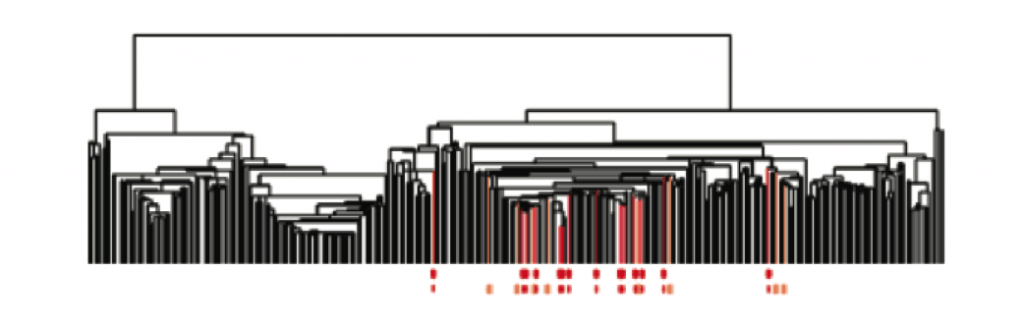Here the parts of the dendrogram that correspond to members of the “perfect survival group” mentioned above are colored in red. It is clear that there is a high level decomposition into two groups, and that all the members of the perfect survival group fall into one of those high level groups.

However, there are many non-members of the perfect survival group which lie in the same high level group, and it will be very difficult to identify where they fit within that group, due to the fact that they are dispersed among many of the leaves of the dendrogram. One would have been unlikely to discover them using only the dendrogram, whereas they are quite apparent in the TDA network displayed above.

The fundamental problem is that clustering is a methodology for breaking data sets apart into pieces, and that is its only tool. Once the data set is such that it contains progressions, and is in an appropriate sense “connected”, clustering methods will typically break things apart that belong together, and that phenomenon is exhibited in the above dendrogram.

The basic point is that representing some aspects of geometry beyond the breakup into clusters provides greater insight into the detail of the data set, which is difficult to recover using clustering alone.